• 本文以SSD算法的网络结构为基础,通过添加感受野模块增强轻量级主干网络的特征提取能力,同时引入特征融合模块,充分利用深层网络提取语义信息,达到实时目标检测的目的,同时提高算法整体的检测精度和对小目标的检测能力...
• Q1：感受野指什么？ A1：（1）从局部理解：（上层->本层）感受野等价与卷积核尺寸。  （2）从全局理解：指输出特征层中一个点映射在原始输入图像中的区域。  Tips：通常感受野指全局理解。 Q2：如何计算一个点映射...卷积
• 对图像特征提取中的图像感受野部分进行了大致的概括，主要分为以下几个部分，由感受野的介绍，感受野的作用，增大感受野的方法如池化和空洞卷积，对空洞卷积的原理进行了详细的描述。对图像特征提取中的图像感受野...
• 感受野的计算公式如下：  r1=1,strides=1 rn = rn-1 + (fn – 1)*strides rn表示第n层的感受野 fn表示第n层卷积核大小 striden表示前n-1层stride乘积 striden = strides= stride1*stride2*…*striden-1 这里对...
• 感受野是卷积神经网络里面最重要的概念之一，为了更好地理解卷积神经网络结构，甚至自己设计卷积神经网络，对于感受野的理解是必备的。人工智能 机器学习
• 感受野的概念 在卷积神经网络中，感受野（Receptive Field）的定义是卷积神经网络每一层输出的特征图（feature map）上的像素点在输入图片上映射的区域大小。再通俗点的解释是，特征图上的一个点对应输入图上的区域...
• 能够计算神经网络感受野，算出感受野的大小，还有一些其他的文件都很有用
• 这里写目录标题概念举例感受野的计算规律两种计算感受野的方法从前往后从后往前推导VGG16网络的感受野结构从后往前从前往后感受野大于图片 概念 在卷积神经网络中，感受野（Receptive Field）的定义是卷积神经网络...


目录
概念举例感受野的计算规律两种计算感受野的方法从前往后从后往前
推导VGG16网络的感受野结构从后往前从前往后
感受野大于图片为什么要增大感受野

概念
在卷积神经网络中，感受野（Receptive Field）的定义是卷积神经网络每一层输出的特征图（feature map）上的像素点在输入图片上映射的区域大小。再通俗点的解释是，特征图上的一个点对应输入图上的区域，如图1所示。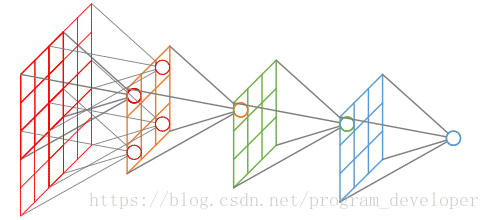在典型CNN结构中，FC层每个输出节点的值都依赖FC层所有输入，而CONV层每个输出节点的值仅依赖CONV层输入的一个区域， 这个区域之外的其他输入值都不会影响输出值，该区域就是感受野。
举例
两层3*3的卷积核卷积操作之后的感受野是5*5，其中卷积核（filter）的步长（stride）为1、padding为0，如图所示：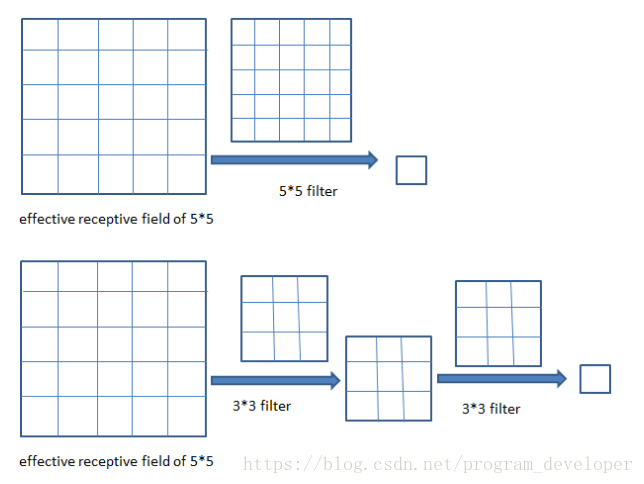三层3*3卷积核操作之后的感受野是7*7，其中卷积核的步长为1，padding为0，如图所示：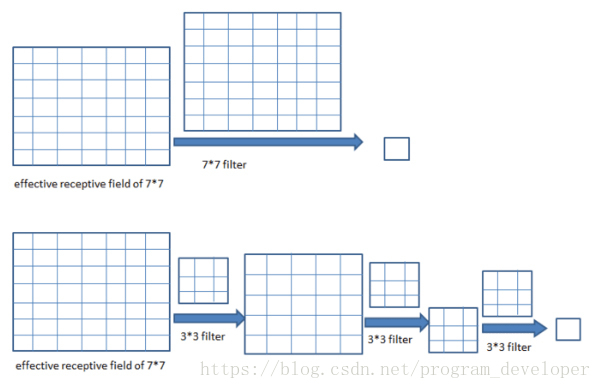感受野的计算规律
设感受野为r（即r为一个元素映射到输入图像的正方形矩阵的边长，这里假设卷积核为正方形，所以映射也是正方形），s为步长，s1表示步长=1，s2表示步长=2，k为卷积核尺寸。初始feature map的感受野是1，即

r

0

=

1

r_{0}=1

，很明显嘛，输入图像还没有经过任何操作，每个元素的感受野当然是1。规定初始s是1，即

s

0

=

1

s_{0}=1

。每经过一个conv k*k s，感受野按照如下公式变化：

r

i

+

1

=

r

i

+

(

k

i

+

1

−

1

)

∗

∏

n

=

0

i

s

n

r_{i+1}=r_{i}+(k_{i+1}-1)*\displaystyle\prod_{n=0}^{i} s_{n}

。 一定要注意这个公式最后的连乘，我自己推公式的时候以为不是连乘而是

s

i

s_{i}

，其实是是不对的。因为很多博客的推导感受野公式的思路和我不一样，直到看到这篇博客是用的连乘我才意识到自己推导的公式的错误所在。博客链接：https://www.cnblogs.com/ocean1100/p/9864193.html由上述公式可知，

r

1

=

k

1

r_{1}=k_{1}

必然成立，也就是说，第一层的感受野必然等于卷积核的尺寸，这与已知符合。池化操作和卷积操作都对应上面的公式，其实仔细想想，池化和卷积其实是很相似的。上述公式是从前往后计算感受野的公式，而从后往前计算感受野可以用下面这个公式：

r

i

−

1

=

(

r

i

−

1

)

∗

s

i

−

1

+

k

i

−

1

r_{i-1}=(r_{i}-1)*s_{i-1}+k_{i-1}

此时最后一层感受野的大小=卷积核的大小，其实这种方法的原理就是倒过来看问题，你想最后一层感受野就像是细胞，模拟细胞分裂的过程，

+

k

i

−

1

+k_{i-1}

其实是从

r

i

r_{i}

中取出1来，这个细胞其实是由前一层卷过来的，卷积核尺寸是

k

i

−

1

k_{i-1}

，所以要加

k

i

−

1

k_{i-1}

，而

剩

下

的

r

i

剩下的r_{i}

，即

r

i

−

1

r_{i}-1

个感受野则是对应步长的。其实文字不太好叙述，大家需要好好理解一下，从后往前的好处就是不用计算s的叠乘了，不过这对于计算机来说并不是什么难事，从后往前是一种反向思维，我个人感觉从前往后更好理解一些。特殊情况，经过conv1x1 s1不会改变感受野，经过FC层和GAP层，感受野就是整个输入图像经过多分枝的路径，按照感受野最大支路计算，shotcut也一样所以不会改变感受野ReLU, BN，dropout等元素级操作不会影响感受野全局步进等于经过所有层的步进累乘，即

∏

n

=

0

i

s

n

\displaystyle\prod_{n=0}^{i} s_{n}

f

o

u

t

=

(

f

i

n

−

k

+

2

∗

p

)

/

s

+

1

f_{out}=(f_{in}-k+2*p)/s+1

反推出P即可
两种计算感受野的方法
从前往后
我们来计算一个简单的例子，先学会计算感受野，再来分析复杂的网络。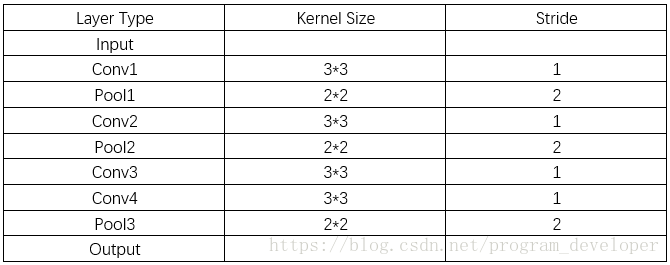根据上面的规律，我们可以计算：

r

0

=

1

，

s

0

=

1

r_{0}=1，s_{0}=1

r

1

=

3

r_{1}=3

r

2

=

3

+

(

2

−

1

)

∗

1

=

4

r_{2}=3+(2-1)*1=4

（r2即对应pool1层）

r

3

=

4

+

(

3

−

1

)

∗

2

=

8

r_{3}=4+(3-1)*2=8

r

4

=

8

+

(

2

−

1

)

∗

2

=

10

r_{4}=8+(2-1)*2=10

（r4即对应pool2层）

r

5

=

10

+

(

3

−

1

)

∗

4

=

18

r_{5}=10+(3-1)*4=18

r

6

=

18

+

(

3

−

1

)

∗

4

=

26

r_{6}=18+(3-1)*4=26

（r6即对应conv4层）

r

7

=

26

+

(

2

−

1

)

∗

4

=

30

r_{7}=26+(2-1)*4=30

从后往前
我们从最后一层的池化层开始计算感受野：

p

o

o

l

3

：

R

=

2

（

最

后

一

层

池

化

层

输

出

特

征

图

的

感

受

野

大

小

等

于

卷

积

核

的

大

小

）

pool3：R=2（最后一层池化层输出特征图的感受野大小等于卷积核的大小）

c

o

n

v

4

：

R

=

（

2

−

1

）

∗

1

+

3

=

4

。

conv4：R=（2-1）*1+3=4。

c

o

n

v

3

：

R

=

（

4

−

1

）

∗

1

+

3

=

6

。

conv3：R=（4-1）*1+3=6。

p

o

o

l

2

：

R

=

（

6

−

1

）

∗

2

+

2

=

12

。

pool2：R=（6-1）*2+2=12。

c

o

n

v

2

：

R

=

（

12

−

1

）

∗

1

+

3

=

14

。

conv2：R=（12-1）*1+3=14。

p

o

o

l

1

：

R

=

（

14

−

1

）

∗

2

+

2

=

28

。

pool1：R=（14-1）*2+2=28。

c

o

n

v

1

：

R

=

（

28

−

1

）

∗

1

+

3

=

30

。

conv1：R=（28-1）*1+3=30。

推导VGG16网络的感受野
结构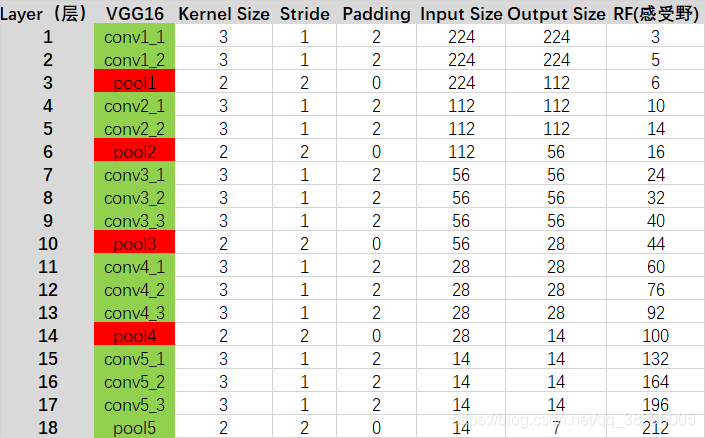从后往前
在VGG16中：pool5中 pool5:RF=2 conv5_3 ：RF=(2-1)*1+3=4 conv5_2 : RF=(4-1)*1+3=6 conv5_2: RF=(6-1)*1+3=8 conv5_1: RF=(8-1)*2+2=16 pool4 : RF=(8-1)*2+2=16 conv4_3: RF=(16-1)*1+3=18 conv4_2: RF=(18-1)*1+3=20 conv4_1 : RF=(20-1)*1+3=22 pool3: RF=(22-1)*2+2=44 conv3_3: RF=(44-1)*1+3=46 conv3_2: RF=(46-1)*1+3=48 conv3_1: RF=(48-1)*1+3=50 pool2: RF=(50-1)*2+2=100 conv2_2: RF=(150-1)*1+3=152 conv2_1: RF=（152-1)*1+3=154 pool1: RF=(154-1)*2+2=208 conv1_2: RF=(208-1)*1+3=210 conv1_1: RF=(210-1)1+3=212
从前往后
conv1_1: RF=3 conv1_2: RF=3+(3-1)*1=5 pool1: RF=5+(2-1)*1=6 conv2_1: RF=6+(3-1)*2=10 … 后面的数在上图的栏目里都有了，我就不一一计算了，最终结果也是212
感受野大于图片
为什么要增大感受野
卷积、池化都有一个重要作用就是增大感受野，为什么感受野这么重要呢？前面看过感受野的定义，是表示在前几层对应的图像区域的大小，那么为了保证所利用的信息是全局的，而不仅仅是局部信息，我们就应该保证感受野较大。同时，合理计算感受野，可以保证效率，例如，输入图像大小是128*128，如果最后一层的感受野已经可以超过128，我们可以认为在做最后的分类判断时所用到的特征已经涵盖了原始图像所有范围的信息了。如果能够尽可能的降低网络的参数总量，也十分重要。
展开全文卷积 计算机视觉
• 针对轻量级深度神经网络MobileNet会减少分类准确率的问题，将空洞卷积核引入MobileNet模型的某一卷积层中，提出一种基于局部感受野扩张的D-MobileNet模型。该模型根据空洞卷积核所在位置的不同分为三种结构，在不...
• 浅层特征图不检测大目标是因为感受野不够，所谓感受野，就是用来表示网络内部的不同位置的神经元对原图像的感受范围的大小。只有当感受野大于物体，能够将物体包含的时候，才能正确检测。在深层特征图，感受野比较大...
之前在做物体检测的时候，对这个问题没有弄清楚，记录下最近关于这个问题的理解。
问题：浅层特征图检测小目标，深层特征图检测大目标？
浅层特征图不检测大目标是因为感受野不够，所谓感受野，就是用来表示网络内部的不同位置的神经元对原图像的感受范围的大小。只有当感受野大于物体，能够将物体包含的时候，才能正确检测。在深层特征图，感受野比较大，可以更好的看清一个物体，用于检测大目标准确度更高。浅层特征图感受野小，对于大物体不能够获取全部信息，比如检测一只动物，却只能检测到一条腿，通过腿去判断动物的类别，就可能会产生误判。由于监督学习模式，越深的层得到的特征越抽象（高级），越浅的层特征越细节（低级）。深层的特征由于平移不变性（translation invariance）,已经丢掉了很多位置信息。检测既需要分类也需要回归。分类要求特征有较多的高级信息，回归（定位）要求特征包含更过的细节信息，因此，大的特征图由于感受野较小，同时特征包含位置信息丰富，适合检测小物体。
感受野计算公式：

l

(

k

)

=

(

l

(

k

−

1

)

−

1

)

+

[

f

(

k

)

∗

∏

i

=

1

k

−

1

s

i

]

l_{(k)} = (l_{(k-1)}-1)+[f(k)*\prod_{i=1}^{k-1}s_i]

l

k

l_k

：第k层的感受野

f

k

f_k

：第k层的kernel_size

s

k

s_k

：第k层的stride
参考资料：
卷积神经网络的感受野 如何计算感受野(Receptive Field)——原理 目标检测中的Anchor
展开全文深度学习
• 通过模拟生物的视觉系统，实现对图片的滤波得到物体的轮廓特征
• 用于准确和快速对象检测的感受野块网络 作者：刘松涛、黄帝、王云红 更新 (2021/07/23)：YOLOX 来 ，更强大的 YOLO，支持 ONNX、TensorRT、ncnn 和 OpenVino！ 更新：我们提出了一种在 COCO 上以 45 FPS 获得 42.4 ...
• 文章目录感受野增加感受野有效感受野与反卷积的区别总结 感受野 卷积核的大小(高度和宽度)定义了一个区域的空间范围，改区域可以被卷积核在每个卷积步骤中修改，因而卷积核的大小称为卷积核的“感受野”。 感受野...


文章目录
感受野增加感受野有效感受野与反卷积的区别总结

感受野
卷积核的大小(高度和宽度)定义了一个区域的空间范围，改区域可以被卷积核在每个卷积步骤中修改，因而卷积核的大小称为卷积核的“感受野”。 感受野(receptive field, RF)，卷积神经网络每一层输出的特征图（feature map）上的特征点在原始图像上映射的区域大小，即特征点能“看”到的范围。越高（深）层的特征点描述的原图信息越全面，越能表述语义信息。
神经元之所以无法对原始图像的所有信息进行感知，是因为在卷积神经网络中普遍使用卷积层和pooling层，在层与层之间均为局部连接。
神经元感受野的值越大表示其能接触到的原始图像范围就越大，也意味着它可能蕴含更为全局，语义层次更高的特征；相反，值越小则表示其所包含的特征越趋向局部和细节。因此感受野的值可以用来大致判断每一层的抽象层次。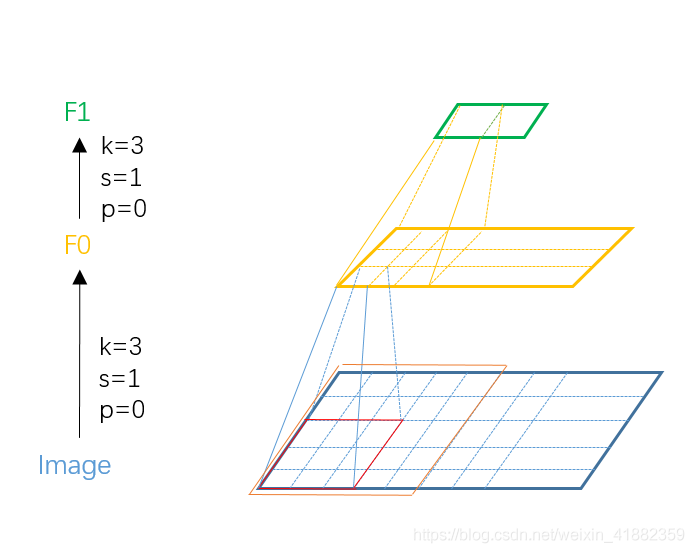感受野计算公式：
其中，L 表示感受野大小，f 表示卷积核(kernel size)，s 表示卷积步长(stride)。起始卷积层(F0)，感受野大小等于。

l

k

=

f

+

n

(

f

−

1

)

l_k=f+n(f-1)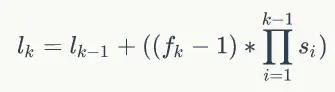其中，

f

k

f_k

表示第n层的卷积核大小，

s

i

s_i

表示每一个前一层的步幅长度，

l

k

−

1

l_{k-1}

表示前一层的有效感受野。
对于上图，计算上图感受野： F0层: L0 = f = 3; F1层: L1 = 3 + (3-1) * 1 = 5; 特别的，当前特征层(feature map)感受野与之前层相关，与当前层卷积核大小相关，与步长(stride),填充(padding)无关。理论感受野尺寸可以大于网络输入尺寸。
增加感受野
为了实现具有相对减少的参数数量的非常深的模型，成功的策略是将许多具有较小的感受野的卷积层堆叠。但是限制了所学习的卷积核的空间大小，其仅与层数线性地成比例。
扩张卷积(空洞卷积) 是一种扩展感受野大小而不增加参数数量的方法。中心思想是引入新的空洞参数(d)，其在执行卷积时决定卷积核权重之间的间隔。一个因子为d的空洞意味着原始卷积核在每个元素之间扩展d-1个空格，并且中间的空位置用零填充。 将尺寸为fxf的卷积核放大到大小为f+(d-1)(f-1).对应于具有预定义卷积核大小f、零填充幅度p、步幅s、空洞因子d且高度h和宽度w的输入的卷积运算的输出维度如下

h

‘

=

(

h

−

f

−

(

d

−

1

)

(

f

−

1

)

+

s

+

2

p

)

/

s

h^={(h-f-(d-1)(f-1)+s+2p)/s}

w

‘

=

(

w

−

f

−

(

d

−

1

)

(

f

−

1

)

+

s

+

2

p

)

/

s

w^={(w-f-(d-1)(f-1)+s+2p)/s}

第n层的有效感受野可以表示为：

R

F

n

=

R

F

n

−

1

+

d

(

f

−

1

)

RF^n=RF^{n-1}+d(f-1)

，满足

R

F

1

=

f

RF^1=f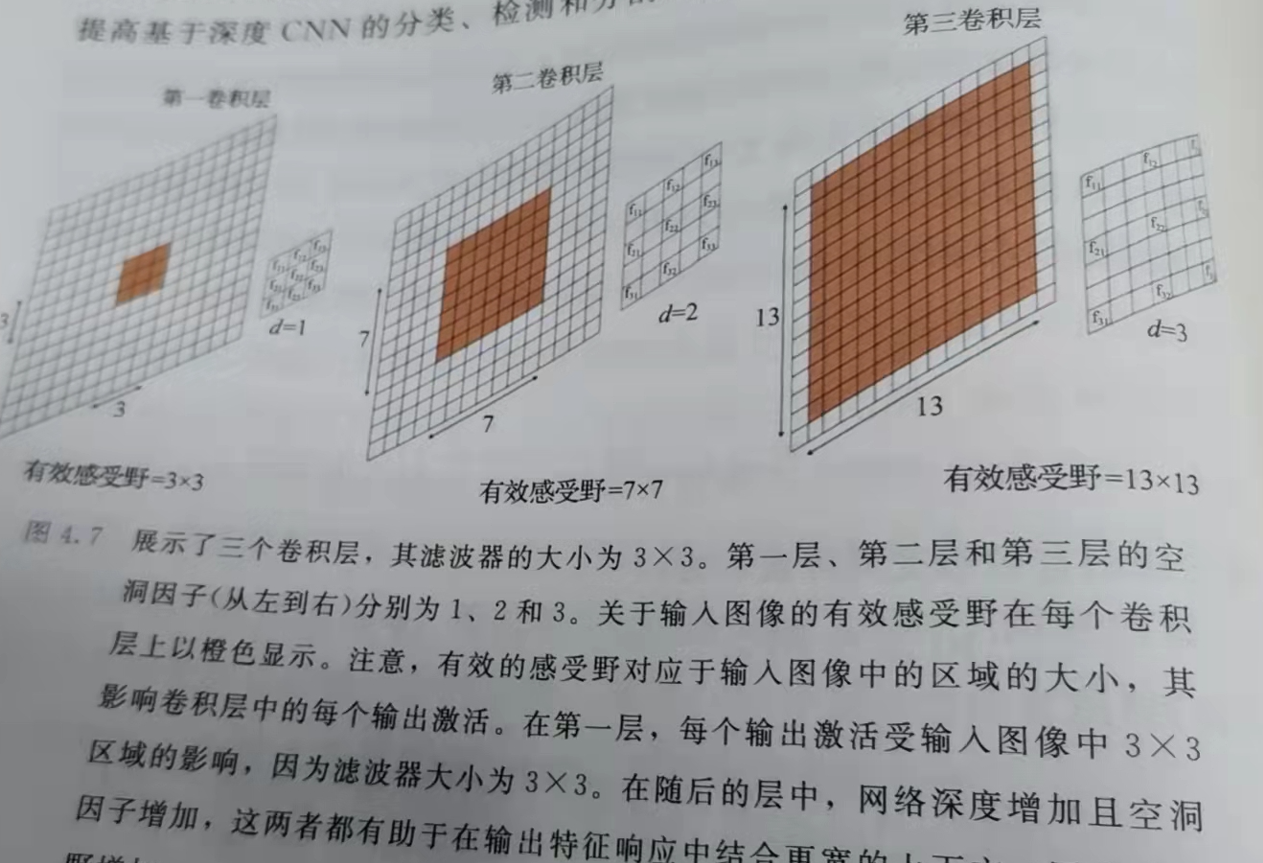1）增加网络层数。特别的，增加网络层数时，对于 f!=1 的网络层数即使feature map大小没有改变，感受野也会增加。 2）增加池化层。成倍的增加感受野。 3）更大的卷积核。通常为了保持feature map的尺寸，更大的卷积核需要相应的padding作为补充。例如，卷积核(k=3,s=2,p=0)与(k=7,s=2,p=3)能够获得相同尺寸的feature map，但后者的感受野要大于前者。
有效感受野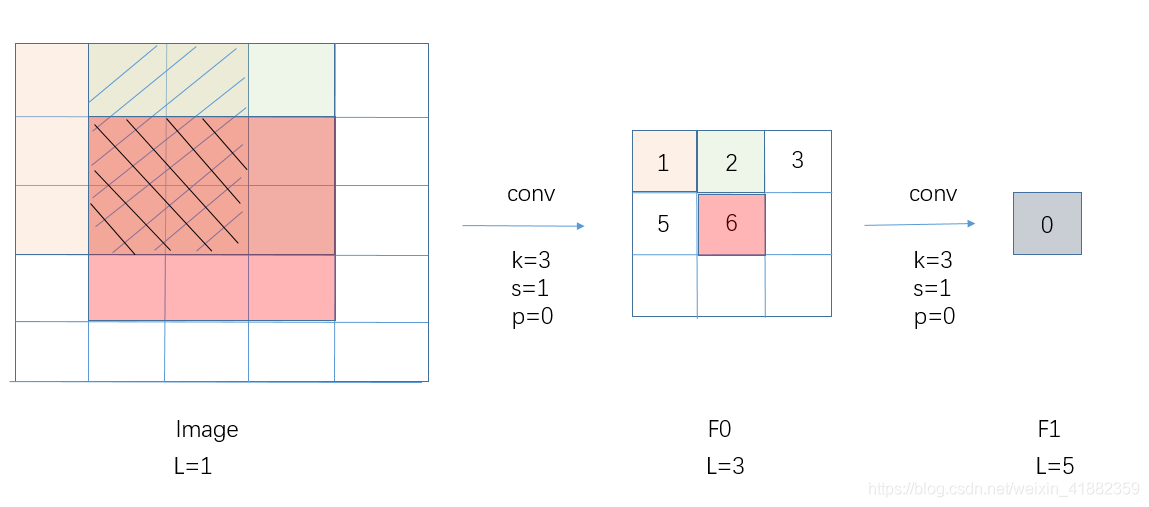有效感受野(effective receptive field, ERF)，在卷积计算时，实际有效的感受野区域。在F0特征层中，特征点6可以描述其他所有特征点的部分信息，即图中交叠部分，特征点6代表的信息更“有效”。即越靠近感受野中心的区域越有效。在网络训练时，有效感受野对参数的影响更大。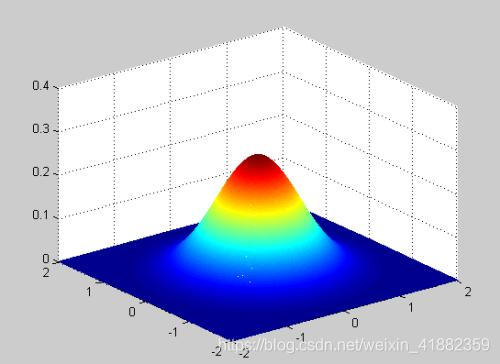与反卷积的区别
反卷积（deconv）： 主要用于增大图像尺寸，是upsampling的一种，而空洞卷积并没有做upsampling，空洞卷积是为了增大感受野，可以不改变图像的大小。 对于反卷积简单理解就是在输入特征矩阵中插入空白点，再进行卷积，这样卷积后输出的特征矩阵就变大了。
对于标准k*k卷积核，stride为s，分三种情况：
上面3）所描述的就是反卷积，它的核心是在原来图像上插入空白数据。而空洞卷积就是在卷积核插入空白数据，或是说在卷积是跳过特征图的部分数据。
总结
神经网络中，感受野可以描述特征点的最大信息量，有效感受野则可以描述信息的有效性。充分理解感受野与有效感受野的概念，在设计网络时，可以依据此计算网络层数，卷积核大小，卷积步长。甚至根据任务不同，自动生成backbone。
展开全文深度学习 计算机视觉 人工智能
• 文章主要贡献有以下几点： 并不是感受野内所有像素对输出向量的贡献相同，实际感受野是一个高斯分布，有效感受野仅占理论感受野的一部分 以上就是不同层下的不同的感受野。 使用不同的激活函数与不同的卷积采样方法...
1. 概念
经典的目标检测如Faster R-CNN, YOLOv3等都用到了Anchor, 怎么设计Anchor每个目标检测方法各不相同。Faster R-CNN中的Anchor有三种形状，三种长宽比，比如形状有[128, 256, 512]三个，长宽比有[1:1, 1:2, 2:1]三种，这样组合就是9个anchor。YOLOv3中的Anchor是通过K-Means聚类得到的。这些基于anchor的方法的目的是学习一个从Anchor到GT Box的转换函数，下边我们了解一下理论感受野、Anchor、实际感受野三者之间的关系。
先看一张图：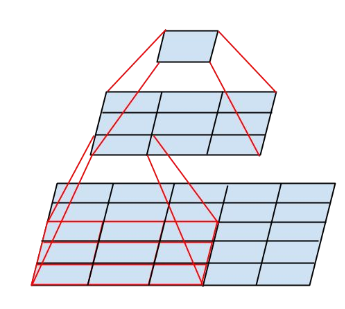图源Medium
一个kernel size=3的卷积核，通过在原feature map上划窗，然后计算得到的是一个值，这个值是通过计算原来feature map上3×3面积上的值得到的结果，那就说经过这个3×3卷积以后，这一层feature map的感受野就是3×3。如果是两个kernel size=3的卷积核堆叠起来，那么上图黄色的feature map每个值对应最下面的5×5的感受野。
**理论感受野：**某一层feature map中的某一个位置，是由前面某一层固定区域输入计算出来的，那这个固定区域就是这个位置的感受野。
实际感受野： 实际感受野要小于理论感受野,是在NIPS2016中的Understanding the Effective Receptive Field in Deep Convolutional Neural Networks提出的。
文章主要贡献有以下几点：
并不是感受野内所有像素对输出向量的贡献相同，实际感受野是一个高斯分布，有效感受野仅占理论感受野的一部分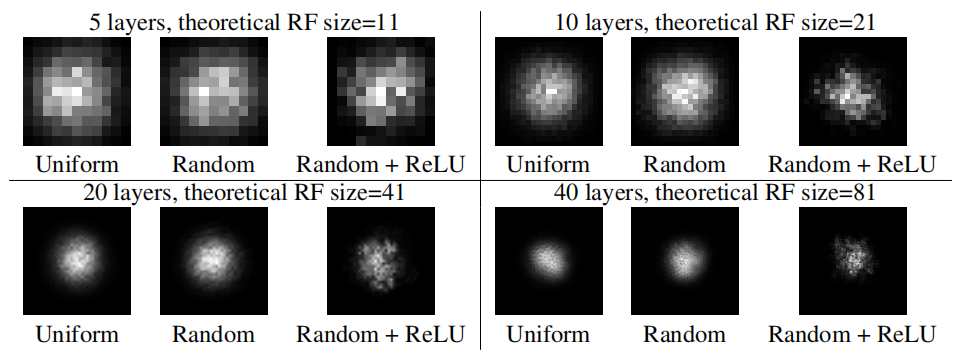以上就是不同层下的不同的感受野。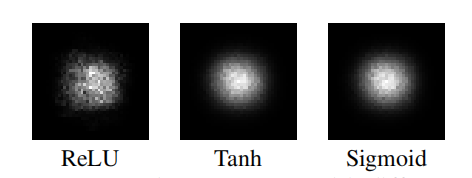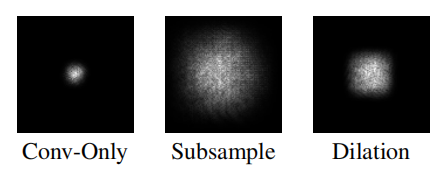使用不同的激活函数与不同的卷积采样方法，产生的实际感受野也不尽相同。其中ReLU的高斯分布没有另外两个平滑，创建了一个较少的高斯分布，ReLU导致很大一部分梯度归零。
另外就是非常关心的问题，实际感受野是如何变化的？实际感受野和理论感受野的关系是什么样的？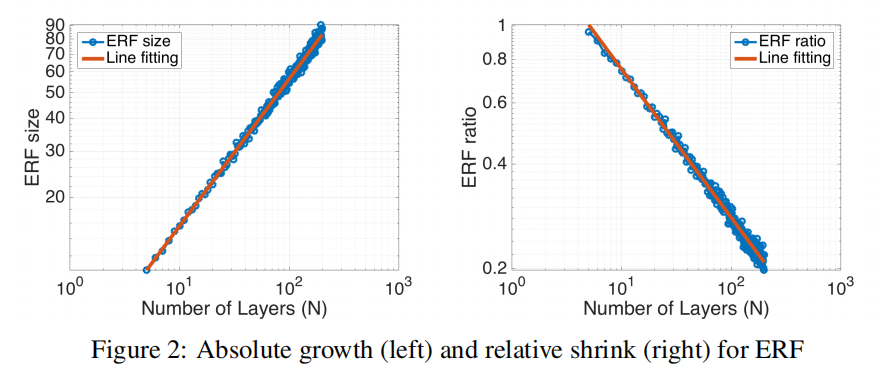文章中也给出了答案，见上图，随着网络层数的加深，实际有效的感受野是程

n

\sqrt{n}

级别增长。而右图展示了随着网络层数的加深，有效感受野占理论感受野的比例是按照

1

/

n

1/\sqrt{n}

级别进行缩减的。其中需要注意的是实际感受野的计算方式：若像素值大于(1-96.45%)的中心像素值，就认为该像素处于实际感受野中。
训练的过程中，感受野也会发生变化。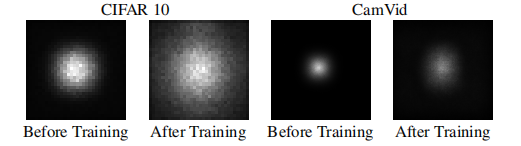可以看出分类和分割任务经过训练后的感受野都有提升，不过提升幅度不太一样。这也说明了神经网络通过学习，扩大了感受也，能够自适应把越来越大的权重放在感受野之外的像素上。也在一定程度上说明更大感受野的必要性。
2. 计算
2.1 一种简单的感受野计算方法
以下内容整理自：zhihu@YaqiLYUhttps://zhuanlan.zhihu.com/p/44106492
规定一下：k代表kernel size, s代表stride, r代表感受野
第一个feature map(也就是原始图片)的感受野默认为1  经过kernelSize=k, s=1 的卷积层, 感受野计算方法如下:

r

=

r

+

(

k

−

1

)

r = r +(k-1)

经过kernelSize=k, s=2 的卷积层, 感受野计算方法如下：

r

=

(

r

×

2

)

+

(

k

−

2

)

r = (r\times2)+(k-2)

经过s=2的maxpool或avgpool层,感受野计算方法如下：

r

=

r

×

2

r = r \times 2

P

=

(

f

o

u

t

−

1

)

×

s

t

r

i

d

e

−

f

i

n

+

k

e

r

n

e

l

S

i

z

e

2

P=\frac{(f_{out}-1)\times stride -f_{in}+ kernelSize}{2}

出个计算题，计算VGG16最后一层感受野, 代入公式：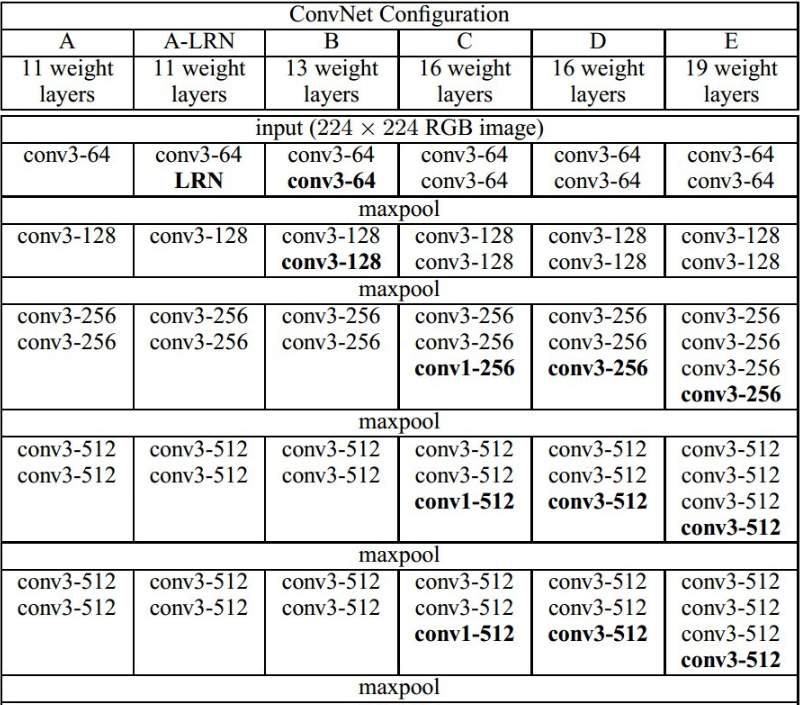VGG16对应D系列，来计算这一列的感受野（从上往下）：
R=(((((1+2+2)x2+2+2)x2+2+2+2)x2+2+2+2)x2+2+2+2)x2=308
S=2x2x2x2x2=32
P=138

实际上tensorflow和pytorch已经有人开发出了计算CNN感受野的模型，实际上VGG16感受野没有那么大，下图是可视化一个416x416大小输入图片的感受野，RF实际上只有212x212,也就是下边黄色和蓝色的正方形。
PS:提供一下pytorch和tensorflow计算感受野的库：
Pytorch:https://github.com/Fangyh09/pytorch-receptive-field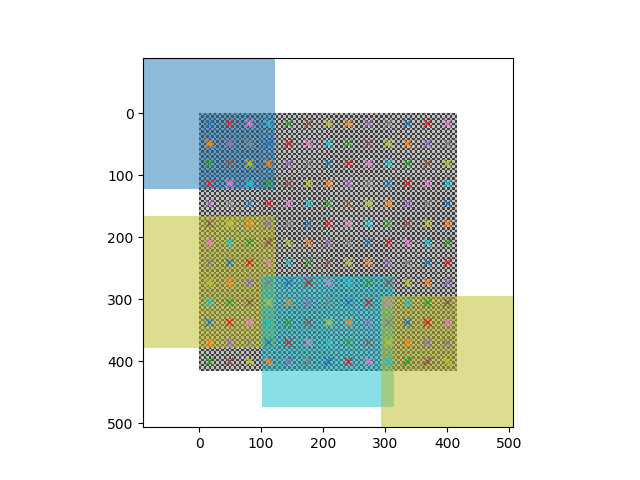以上的计算方式是国内认可度比较高的一种计算方式，在知乎上获得了400+的高赞，但是与谷歌计算出来的结果不一样，所以我就去读了一下谷歌发在Distill上的一篇论文：Computing Receptive Fields of Convolutional Neural Networks，里边非常丰富的讲解了如何计算感受野，包含的情况非常广。先说结论，实际上以上计算方法应该是从下往上进行的，而不是从上往下，另外以上规则只能适合比较有限范围的卷积，而没有一个详细的公式。在讲解下一节以后，我们尝试重新计算VGG的感受野
2.2 自下而上的计算方法
首先也来规定一下默认的符号意义，规定L代表网络的层数

l

=

1

,

2

,

.

.

.

,

L

l=1,2,...,L

, 定义feature map:

f

l

∈

R

h

l

×

w

l

×

d

l

f_l\in R^{h_l\times w_l\times d_l}

, 代表第

l

l

层的输出，高宽深度分别为

h

l

,

w

l

,

d

l

h_l,w_l,d_l

。规定输入的图片为

f

0

f_0

,最后一层feature map输出为

f

l

​

f_l​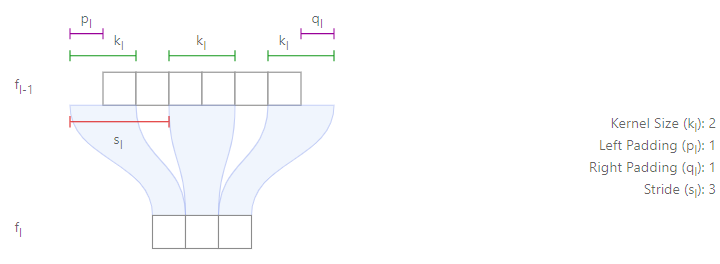l

l

代表第几层；

f

f

代表feature map；

p

p

q

q

s

s

代表stride，

k

​

k​

代表kernel size。
在这里，定义元素级操作的kernel size=1,比如加法，filter concatenation、relu等操作。
下图是一个四层的网络的感受野示意图：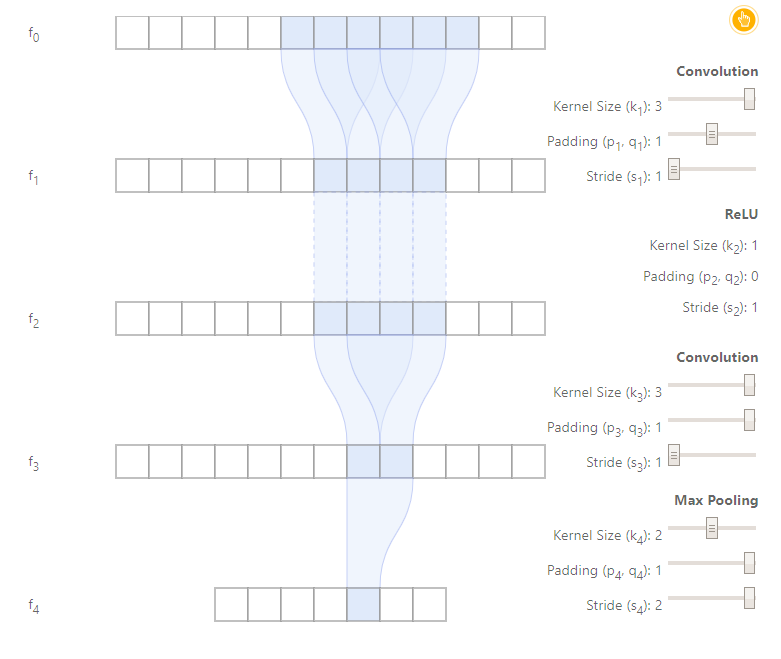经过了两个3x3卷积和一个stride=2的maxpooling层，如果按照原来的方法从上往下进行计算，那最后一层每个点的感受野应该是：(1+2+2)x2=10, 要比上图的6要大很多，所以就知道为啥计算的感受野偏大了（没有处理重叠部分），而且也可以发现感受野中间集中更多信息，两边信息处理较少。
单路网络中的计算方法
单路网络就是没有分支的网络，比如VGG。
规定

r

l

​

r_l​

是第

l

​

l​

层feature map相对于

r

L

​

r_L​

的感受野。说人话，就是最后一层

f

L

​

f_L​

的一个像素值是由第

l

​

l​

层feature map的

r

l

​

r_l​

个值计算出来的，注意

r

L

=

1

​

r_L=1​

。所以整个计算过程是从后往前进行的。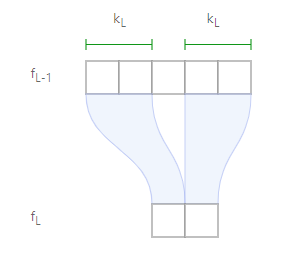上图是一个简单例子，

k

e

r

n

e

l

s

i

z

e

=

2

,

p

a

d

d

i

n

g

=

0

,

s

t

r

i

d

e

=

3

​

的情况下，下边这层每个值只受上一层2个值的影响，所以第

l

−

1

​

l-1​

层的感受野就是2。
然后我们要考虑更加一般的情况，也就是通过

r

l

r_l

能计算出

r

l

−

1

r_{l-1}

,这样就能通过类推，得到最终结果。具体推导过程推荐去原文看一下，非常详细，在这里不多赘述，否则篇幅会太大。直接给出结论：
递推公式:

r

l

−

1

=

s

l

×

r

l

+

(

k

l

−

s

l

)

r_{l-1}=s_l\times r_l+(k_l-s_l)

通项公式:

r

0

=

∑

l

=

1

L

(

(

k

l

−

1

)

∏

i

=

1

l

−

1

s

i

)

+

1

r_0=\sum_{l=1}^{L}((k_l-1)\prod_{i=1}^{l-1}s_i)+1

建议自己推导的时候使用递推公式，非常方便，代码实现的时候考虑使用通项公式。
观察一下通项公式，可以发现，如果每一层kernel size都是1，那么最终的感受野就是1。如果所有的stride=1,那么最终感受野之和就是

∑

(

k

l

−

1

)

+

1

\sum(k_l-1)+1

。并且可以发现，影响感受野最大的参数应该是stride，如果stride>1,那将成倍的影响之后的层。
Effective Stride:
递推公式:

S

l

−

1

=

s

l

×

S

l

S_{l-1}=s_l\times S_l

通项公式：

S

l

=

∏

i

=

l

+

1

L

s

i

S_l=\prod_{i=l+1}^{L}s_i

递推公式：

P

l

−

1

=

s

l

×

P

l

+

p

l

P_{l-1}=s_l\times P_l+p_l

通项公式:

P

l

=

∑

m

=

l

+

1

L

p

m

∏

i

=

l

+

1

m

−

1

s

i

P_l=\sum_{m=l+1}^{L}p_m\prod^{m-1}_{i=l+1}s_i

下面我们来重新计算VGG16整个模型的感受野：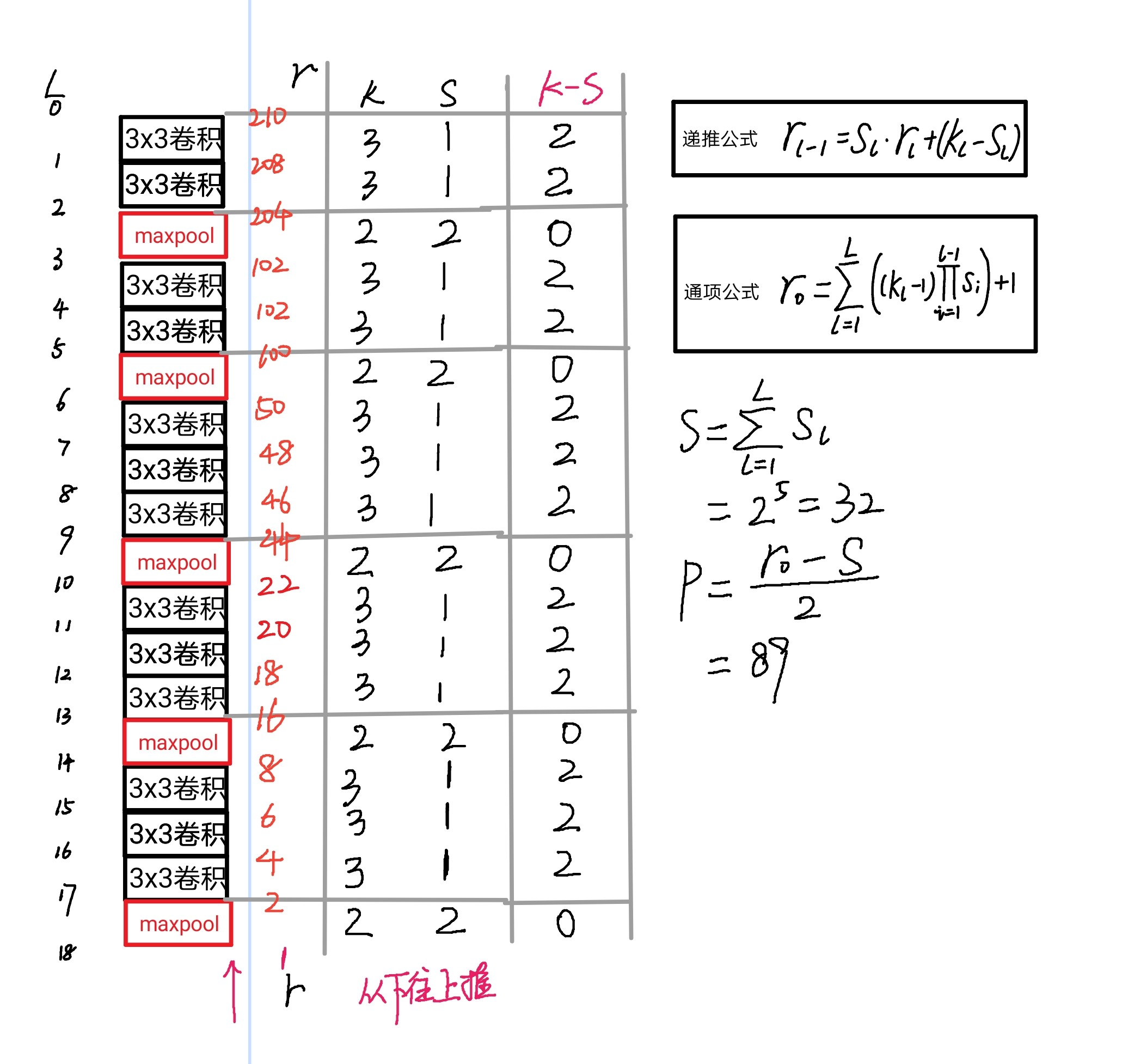多路网络
以上讲解了单路情况下的感受野计算方法，但是现在STOA的卷积网络通常都是拥有多分支的，比如说ResNet、Inception、DenseNet等网络。
多路网络不得不提到对齐问题，感受野是不具有平移不变性，下图就是一个例子: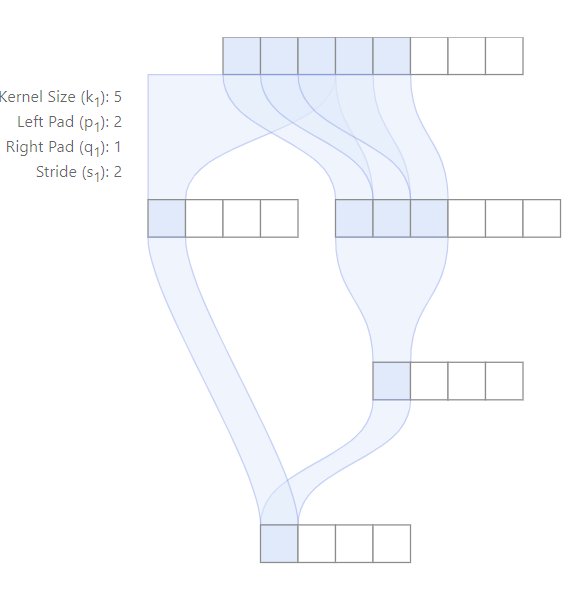可以看到，最后一层通过两条不同路径后对应到第一层feature map的感受野的中心是不重合的。这是感受野的一个性质，不过好在大多数现代的网络设计是对齐的，对很多计算机视觉任务来说，都需要对其输出特征，包括目标检测、语义分割、边缘检测、着色等。
在网络对齐时候，所有不同路径都会导致感受野的中心是重合的，所以不同路径必须保持相同的stride。这一点可以观察Inception系列的网络，两条路的Stride是不可能不同的。
在网络对齐的情况下，多路网络的感受野应该是所有分支中最大感受野，比如下边是一个对齐的网络，中心是重合的，感受野的大小就是3。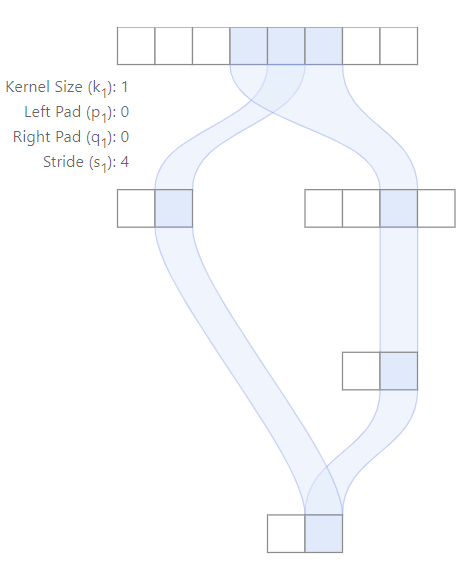有了以上的计算方法，我们可以很方便计算所有卷积网络的感受野：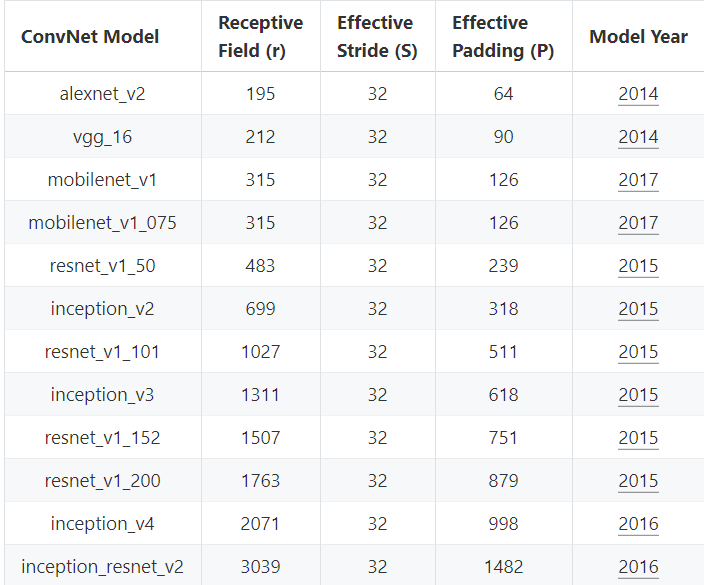上图是常用分类模型对应的感受野的结果，我们可以发现，随着模型的不断进化，感受野在不增大，在比较新提出的网络中，感受野已经能够覆盖整个输入图像了，这就意味着最终特征图中每个点都会使用到整个图像所有得上下文信息。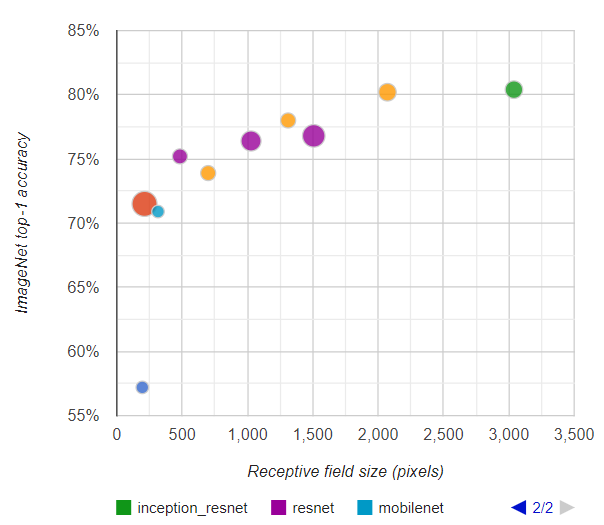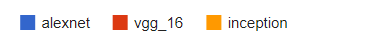上图是感受野大小和在ImageNet上top-1准确率的关系，其中圆圈的大小代表每个网络的浮点运算数量。分类的准确率和感受野大小大体程对数关系，也就是说虽然感受野可以无限增长，越往后，带来的准确率上的提升也就越小，而需要的计算代价会变得更大。
上图MobileNet和VGG16准确率相当，但是MobileNet所需计算量却非常小（约为VGG16的27分之1），这是由于MobileNet使用了大量depth-wise Convolution,这样可以以较小的计算代价来增加模型感受野。这个对比可以表明生成更大感受野的网络可以有更好的识别准确率。
注意: 感受野并不是唯一影响准确率的因素，其他因素，比如网络的深度，宽度，残差连接，BatchNorm等也起着非常重要的作用。也就是说，感受野是我们在设计网络的时候需要考虑的一个因素，但还是要综合其他方法。
补充：
除了最普通的卷积，还有空洞卷积、上采样、BatchNorm、Separable Conv的感受野计算需要补充。
空洞卷积：引入空洞率

α

\alpha

, 经过空洞卷积，kernel size有原来的

k

k

变为

α

×

(

k

−

1

)

+

1

\alpha \times (k-1)+1

,所以只要替换原来的公式中的k即可。上采样：一般通过插值来实现，假如

α

\alpha

通过2倍上采样得到

β

\beta

，那么认为这个层感受野计算等价于与

α

\alpha

的kernel size相等的卷积。(这个地方不是特别理解，按照自己想的，一般upsample不会改变感受野，下边是谷歌论文中关于这部分的讲解，希望能读懂的大佬能联系一下我，我的联系方式在文末)

Upsampling. Upsampling is frequently done using interpolation (e.g., bilinear, bicubic or nearest-neighbor methods), resulting in an equal or larger receptive field — since it relies on one or multiple features from the input. Upsampling layers generally produce output features which depend locally on input features, and for receptive field computation purposes can be considered to have a kernel size equal to the number of input features involved in the computation of an output feature.

可分离卷积: 等价于普通卷积，并没有变化。BatchNorm:推理过程中BN不改变感受野，而训练过程中，BN的感受野是整个输入图像。转置卷积: 会增加感受野，和普通计算方法类似
3. 作用
1.目标检测：像SSD、RPN、YOLOv3等都使用了anchor，而anchor的设计正是依据感受野，如果感受野太小，只能观察到局部的特征，不足以得到整个目标的信息。如果感受野过大，则会引入过多噪声和无效信息。Anchor太大或太小均会影响性能。
2.**语义分割：**最终预测的像素的感受野越大越好，涉及网络一般也是越深越好，这样才能捕获更多的上下文信息，预测才会更准。
3.**分类任务：**图像分类中最后卷积层的感受野要大于输入图像，网络深度越深，感受野越大，性能越好。
为了更合适的感受野，各种多尺度的模型架构被提出，可以分为图像金字塔和特征金字塔，具体实现方面可以是：1. 采用多尺度输入，如yolov3的multi scale机制。2. 多尺度特征融合，最经典的是FPN。3. 多尺度特征预测融合，如SSD。4. 以上方法的多种组合。
4. 关系
在论文 Single Shot Scale-invariant Face Detector 中，说明了目标检测中Anchor, 理论感受野，实际感受野三者的关系。这篇论文主要针对的是中小目标，密集人脸情况下的anchor设置。
Anchor一般是通过先验进行指定的，Anchor与目标的大小和位置越匹配(IOU>0.5or0.7)，回归效果就会越好。如果小人脸和anchor的IOU过小，就会造成anchor很难回归到GT上，从而导致Recall过低。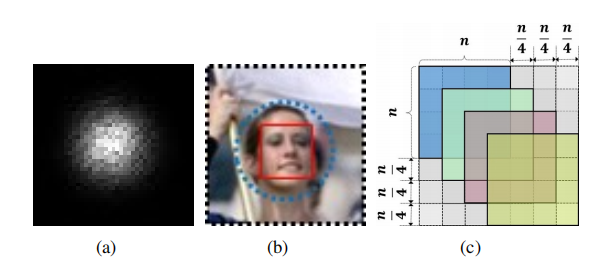文章中指出了Anchor、理论感受野、实际感受野三者的关系。（a）图中，整个黑色的box是理论感受野，中间的白点是一个高斯分布的实际感受野。（b）图中举了一个例子，黑色点状的框代表是理论感受野的面积，蓝色的代表实际感受野位置，而最合适的anchor就是红色的框，所以关系为：
Anchor大小<实际感受野<理论感受野
SFD是基于SSD进行改进的，SSD中使用了6个层来进行检测，以下是文章中设置内容: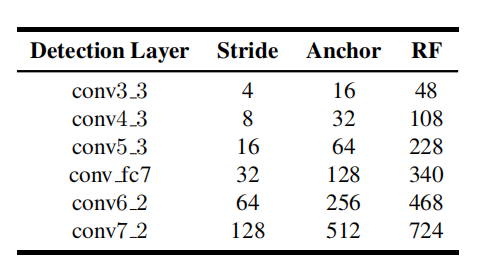为了探究Anchor和RF的关系，这里使用MATLAB的工具箱来拟合两者关系：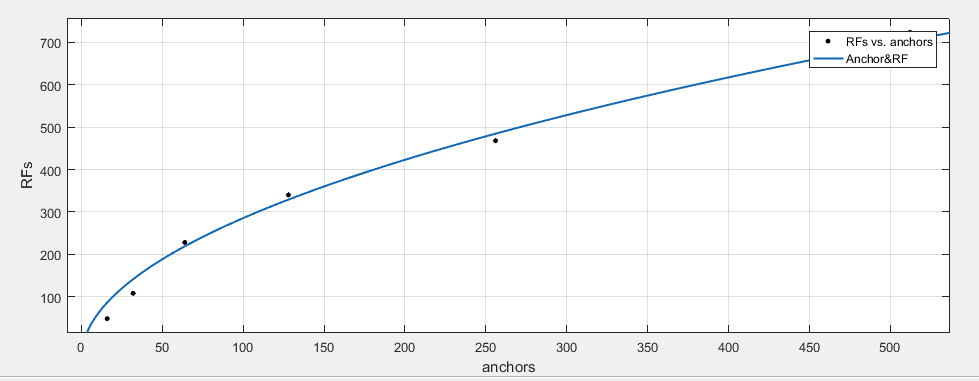设anchors=x, RFs=y则有如下关系：

y

=

9.421

×

12.44

×

x

−

46.92

R

2

=

0.989

y=9.421\times \sqrt{12.44\times x}-46.92 \\ R^2=0.989

所以y和

x

​

\sqrt{x}​

大体上是正比例关系。其实这里只是探索一下他们之间的关系，实际感受野实际上依然是一个超参数，我们不可能明确计算出来，然后设置对应的anchor，不过我们了解了这个之后，最起码anchor的限制范围应该是小于理论感受野的。
作者在论文中说明了Anchor设置的理由，

A

n

c

h

o

r

=

s

t

i

r

d

e

×

4

Anchor=stirde\times 4

, 这样就跟上（c）图一样，这样设置可以保证不同尺度的anchor在图片上采样的密度是一样的。将铺设的anchor的scale值设为大致覆盖到有效感受野的size。这一点并不是特别理解
SFD效果可以说是非常好了，以下是mAP对比图：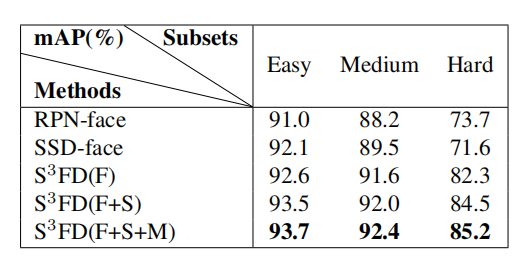5. 总结
首先，许多经典的网络结构中的这些卷积不是随便决定的，在修改网络的时候不能随便删除。比如在yolov3中，backbone后边的几个卷积再接yolo层这个当时就觉得很随意，但是他是有一定道理的。  假如出于对小目标的考虑，想要降低下采样率，那么直接删掉一个maxpool层，或者删掉stride=2的卷积层都是不可取的。通过公式我们知道，stride对一个网络的感受野影响非常大，而且会对后边的网络层的感受野都进行影响，所以要通过使用stride=1的maxpool或者空洞卷积来弥补对感受野带来的损失。  实际感受野依然是一个超参数，他是会随着训练的过程发生变化，我们无法准确计算出来实际感受野，但是通过分析anchor，实际感受野和理论感受野，我们知道了anchor<实际感受野<理论感受野，所以anchor还是会被理论感受野的大小所限制。  自己尝试过使用kmeans算法聚类自己数据集的Anchor，之前两个数据，两次聚类，都出现了自己聚类得到的Anchor不如默认的Anchor得到的结果。之前一直不知道原因在哪里。在总结了感受野以后，我觉得可以合理推测，Anchor应该和实际感受野尽量匹配，但是实际感受野实际上是一个超参数，通过聚类得到的anchor有一定代表性，但是效果反而不如默认的anchor，这是因为我们自己聚类得到的Anchor的范围实际上没有默认的广。 比如yolov3-tiny里边的anchor最小是【10，14】最大是【344，319】,经过计算理论感受野是382,按照SFD里边推导得到的公式计算，对应的anchor大小应该为159，如果按照理论感受野的三分之一来计算，大概是127.3。大概在这个范围，自己聚类得到的最大anchor也就是20左右，所以网络如果想要适应（回归）20左右的anchor需要网络更长时间的训练。
最后想推荐一下以上涉及到的三篇文章，都特别有价值，值得多看几遍：
（1）S3FD
（2）Computing Receptive Fields of Convolutional Neural Networks
（3）Understanding the Effective Receptive Field in Deep Convolutional Neural Networks
另外，以上内容是基于论文以及自己的理解表述的，如果有问题，欢迎加我微信，互相交流。
6. 参考文献
SFD论文: https://arxiv.org/abs/1708.05237
YaqiLYU:https://zhuanlan.zhihu.com/p/44106492
感受野计算论文:https://distill.pub/2019/computing-receptive-fields/
感受野推导: “Computing Receptive Fields of Convolutional Neural Networks”, Distill, 2019.
一个用来计算感受野的网站：https://fomoro.com/research/article/receptive-field-calculator
展开全文计算机视觉 神经网络
• 本文介绍感受野及其计算方法，和有效感受野概念 什么是感受野? 感受野与视觉 感受野(receptive field)这一概念来自于生物神经科学，是指感觉系统中的任一神经元，其所受到的感受器神经元的支配范围。感受器神经元...深度学习 tensorflow pytorch 神经网络 机器学习
• 1. 概念 经典的目标检测如Faster R-CNN, YOLOv3等都用到了Anchor, 怎么设计Anchor每个目标检测方法各不...这些基于anchor的方法的目的是学习一个从Anchor到GT Box的转换函数，下边我们了解一下理论感受野、Anchor、实
• 在卷积神经网络中，感受野是一个非常重要的概念，今天，我们具体来看一下感受野的相关概念以及如何计算感受野感受野 让我们快速回顾一下如何计算感受野的大小。 为了更好地理解感受野，我们可能希望以不同的...
• 感受野是卷积神经网络（CNN）重要概念之一，它起源于人类神经系统，后随神经网络模型在计算机视觉（CV）领域的应用引入到计算机视觉领域。在卷积神经网络占领CV领域绝对地位之后，感受野成为CV领域研究中最重要的...深度卷积神经网络
• 通过学习双目感受野特性对立体图像进行全参考质量评估研究论文
• ## 感受野 深度理解

千次阅读 多人点赞 2018-11-30 18:20:35
知乎是个好东西，深入理解一些理念，靠博客是不行的。 感受野计算和理解的内容参考自：https://zhuanlan.zhihu.com/p/44106492/https://zhuanlan.zhihu.com/p/40267131 ... 目录 ...二：感受野介...
• 感受野 感受野(receptive field, RF)，卷积神经网络每一层输出的特征图（feature map）上的特征点在原始图像上映射的区域大小。深度学习
• ## 卷积神经网络中感受野的详细介绍

万次阅读 多人点赞 2018-07-08 13:38:22
1. 感受野的概念在卷积神经网络中，感受野（Receptive Field）的定义是卷积神经网络每一层输出的特征图（feature map）上的像素点在输入图片上映射的区域大小。再通俗点的解释是，特征图上的一个点对应输入图上的...神经网络 深度学习 感受域
• ## 感受野（ Receptive Field）

千次阅读 多人点赞 2019-01-21 19:43:36
一、理论感受野 1、定义 感受野被定义为卷积神经网络特征所能看到输入图像的区域，换句话说特征输出受感受野区域内的像素点的影响 convNets(cnn)每一层输出的特征图(feature map)上的像素点在原始图像上映射的...
• 什么是感受野感受野”的概念来源于生物神经科学，比如当我们的“感受器”，比如我们的手受到刺激之后，会将刺激传输至中枢神经，但是并不是一个神经元就能够接受整个皮肤的刺激，因为皮肤面积大，一个神经元...卷积神经网络 深度学习 python pytorch
• 该算法将不同层及不同尺度的特征图以轻量级的方式相融合，下采样层生成新的特征图，通过引入感受野模块，提高网络的特征提取能力，增强特征的表征能力和鲁棒性.和传统SSD算法相比，本文算法在精度上有明显提升，同时......